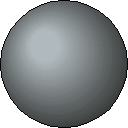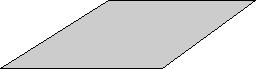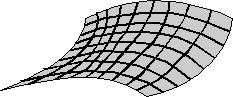## General Relativity and the Possible Geometries for Space

The Friedmann-Robertson-Walker model allows for three possible structures for space: If the mass/energy density of the Universe is more than the critical value then space is a three-dimensional sphere; if the density is equal to the critical value then space is flat; if the density is less than the critical value then space is a hyperbolic three-dimensional sphere. The two-dimensional analogs of the above three spaces are respectively of the surface of a ball, the plane and the saddle surface:Figure 1: Two-Dimensional Sphere
Curvature is positive, Ω > 1, and space is close.Figure 2: Two-Dimensional Plane
Curvature is zero, Ω = 1, and space is flat.Figure 3: A Saddle Surface or Two-Dimensional Hyperbolic Sphere
Curvature is negative, Ω < 1, and space is open.

The ratio of the mass/energy density to its critical value is Ω; Ω determines the geometry of space.
In the case of a sphere, the Universe is closed because it is of finite extension and it turns out that the expansion of the Universe will eventually stop, space will begin to contract instead of stretch and the Universe will finally collapse in a process resembling the opposite of the Big Bang. Hence, for this first structure, the Universe has a finite lifetime. When the Universe is open (the hyperbolic case) or flat, space is of infinite extent and the expansion continues forever.

This page was created by the staff of Jupiter Scientific, an organization devoted to the promotion of science through books, the internet and other means of communication.

This web page and its contents may NOT be copied onto other web sites, but other sites may link to this page.